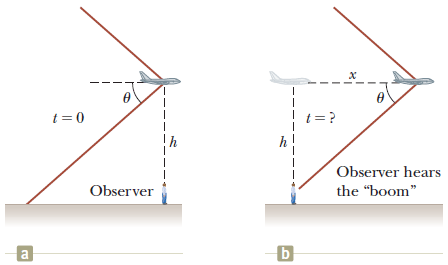# At what time and where will the plane be when this shock is heard

0 like 0 dislike
106 views

A supersonic jet traveling at Mach 2.00 at an altitude of h = 21,000 m is directly over a person at time t = 0 as shown in the figure below. Assume the average speed of sound in air is 335 m/s over the path of the sound.(a) At what time will the person encounter the shock wave due to the sound emitted at t = 0?Reexamine the geometry shown in the diagram to see how to determine time between when the plane passes directly overhead and when the shock wave reaches the observer. s

(b) Where will the plane be when this shock wave is heard? (Give the plane's horizontal displacement x.)asked Dec 15, 2017 in Physics